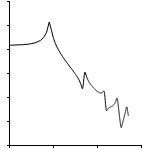WRITTEN BY:

Introduction

Frequency response analysis in Finite Element Analysis (FEA) is used to calculate the steady-state response due to a sinusoidal load applied to a structure at a single frequency. It is a specialized type of transient response analysis that is extremely efficient to solve a very specific type of model.

In the rest of the article, we will briefly introduce the reason for the existence of Frequency Response analysis. We will then derive the equations in as simple a way as possible.

If you are more interested in the use and physical meaning of the analysis type and the interpretation of Frequency Response result, you can skip directly to the last parts of the article.

Why use Frequency Response analyses

Due to the extreme number of calculations, large numbers of time-steps and large results file sizes, Transient analyses are expensive to perform. As an example, time-integrating a structure for 1 minute at a sample rate of 10 kHz with a results-file size of 10 MB per time-step results in 600 000 time-steps and a 6 TB results-file size! Clearly, both the analysis time and post-processing of such a massive results file will be excessive.

Any simplification that can be used to reduce the calculation effort and the results file size is therefore highly appreciated. A modal analysis is one type of simplification where we assume there is no input load. In this case, we’re interested in the characteristics of a structure instead of the response of a structure. For more information on modal analysis and it’s use, see Modal Analysis: What it is and is not

A Frequency Response (FR) analysis is another type of simplification where we assume the input load is applied at a single frequency and that the duration of the load-application is long enough that the response also only occurs at the same frequency. For this type of loading, performing a frequency response analysis can save a lot of time and drive space.

With any simplification there are assumptions. If the assumptions are perfectly met, then the simplification does not impact results accuracy. If we understand the assumptions made for a frequency-response analysis, then we can determine when a frequency response analysis is exact, when it is not exact but useful, and when it is meaningless.

In the rest of this article, we will work through the (surprisingly simple!) mathematics behind a frequency response and explain the meaning and use of the results obtained from a frequency-response analysis.

Deriving the Frequency Response equation

Solving the transient dynamic equation as used in Finite Element Analysis (FEA) requires time-integration of the following equation: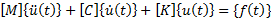In the previous equation,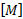,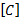and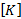are the mass-matrix, viscous damping matrix, and stiffness matrix respectively.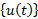,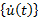and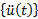are the displacement, velocity and acceleration vectors respectively.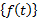is the applied load vector. Note that the displacement and load vectors are time-varying.

This brings us to the first assumption: For frequency response analysis, we assume that the system is linear. Therefore, the stiffness-, mass- and damping-matrices are constant.

The second assumption is that the loadis sinusoidally time-varying at a single frequency. Therefore, we can writeas: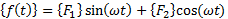In this equation,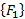and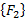are constant vectors, with the only time-varying component the sine and cosine terms, both at the same frequency. The reason for this split to a sine- and cosine-term is that even though the load occurs at a single frequency, the load is not required to be in-phase at all locations where we apply a load. In other words, different locations may have different phase-angles for the applied load.

A different and more convenient way of writingis to use the exponential format: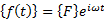In this case,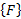is a constant vector with the time-component captured in the term. Phase in this equation is introduced by allowingto be complex.

The third assumption for frequency-response analysis is that the response of the system occurs only at the single input frequency. This may sound “obvious”, but it is entirely possible for a structure with a load at one frequency to have a response at that frequency as well as at any combination of the modes of the system.

The following images show the structural response as an animation as well as a graph of the tip-deflection of a simple beam-structure. The first mode of this beam mesh is at about 8Hz. A sine-wave at 50Hz is applied, starting at 0s with the beam initially in rest. The sudden start of the applied sine-wave load adds energy to the structure over a wide frequency range. Since the 50Hz load is close to the second mode at 51Hz, the deformed shape closely resembles the second mode. However, the first mode at 8Hz is superimposed on the response in both the animation and the graph.Animation of Beam deformation with 50Hz tip load. First mode at 8Hz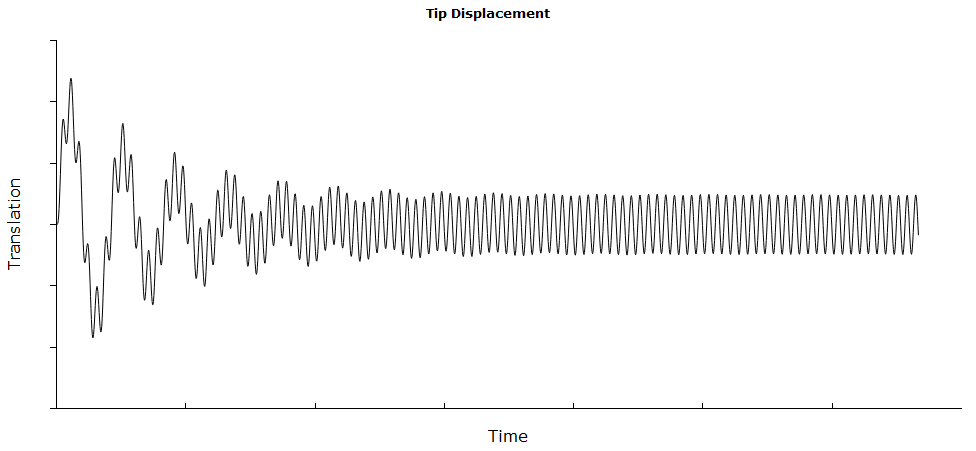Graph showing tip deflection for the animation above. Both 50Hz of the applied load and 8Hz of the first mode is initially present.

Due to the damping in the system, the energy for all modes are damped over time while the continuous input at 50Hz causes a steady state response later in the analysis.

This last part is exactly what we are interested in: The part where the response is purely at the input frequency. This means that the response can be written as: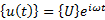In this equation, the steady state displacement is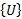, a complex constant vector. Complex because of phase, constant because the steady-state response is not changing. The time-dependent part of the response is again captured purely as a sinewave in the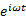term.

The velocity and acceleration are determined from the above equation by taking the first- and second time-derivatives: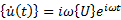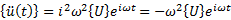We now have enough information to complete the derivation of the Frequency Response form of the dynamic equation: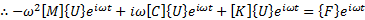To recap, in the previous equation,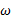is the frequency of the applied load and the response,is the complex load vector andthe complex steady-state response. The reason for the complexandvectors is that each node and direction of the structure can have a load applied with a different phase. Furthermore, the resulting displacement of each node and direction can also have a different phase.

There are times when theterm will be non-zero, in which case the equation can be divided by this term. This results in the following equation: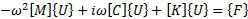Since this form of the equation does not contain any time-dependent behavior, it means that we can directly calculate the steady state response without any form of time-integration. To do this, let’s re-arrange the equation a bit: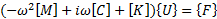Let’s define the term in brackets as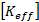, as it represents an “effective stiffness”: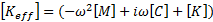In this equation, all terms are known and constant: The frequency of the applied load as well as the mass, damping and stiffness of the structure. Substituting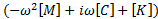with, we can rewrite the Frequency Response equation as: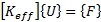The form of this equation is the same as the linear-static analysis equation, meaning it is quick and easy to solve. The only thing that differs is that,andcan all three be complex.

What is a Frequency Response analysis?

A frequency response analysis is used to answer the question:

What is the steady-state response of a linear structure excited at a single frequency?

In other words, it is exactly what a modal analysis is not:

A modal analysis calculates natural frequencies and mode shapes for the case of no load applied – It does not calculate the response of a structure.

On the other hand, a frequency response analysis does calculate the response of a structure. This is a very important distinction between Modal analysis and Frequency Response analysis.

If you’ve read through the derivation above, you’re probably worried that we keep on saying “at a single frequency”. We usually want to know how a structure responds over a range of frequencies.

However, it simply means that each frequency is solved independently, similar to how each time-step in a transient analysis is solved separately. A Frequency Response analysis almost always solves for a number of frequencies in one run. Each is simply calculated completely independently from the others. For each frequency where the Frequency Response was calculated, the results are the steady-state response at that frequency only.

A frequency response analysis is a lot faster to solve with significantly smaller results files compared to a transient response analysis with a lot of time-steps. If the system is linear and we are only interested in the steady-state vibration response, then the answer obtained from a frequency response analysis is exactly what we would have obtained from a transient response that we ran for enough time-steps to reach steady state.

In other words, the analysis is quick to perform and easy to set up. The problem comes in with interpretation of the results. In theory, it is simple, in practice we find it harder to think of a response in the frequency domain than in the time domain.

Notes on damping in Frequency Response analysis

Structural Damping

So far, damping has been assumed to be viscous. For structural damping, we can replace the (velocity-dependent) viscous damping term: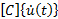with a (displacement-dependent) structural damping term: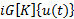This is a displacement-dependent type damping with the structural damping coefficient G. This type of damping can only be approximated in direct transient methods because the complex number which indicates phase has no meaning in transient analysis. However, it can be perfectly represented in frequency response analysis as the term becomes: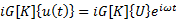The effective stiffness matrix as used in the frequency response calculation changes from:To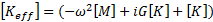Effect of damping on the Frequency Response results

It is entirely possible to solve a frequency-response analysis without damping. The only requirement for frequency response analysis without damping is that the analysis does not take place at a mode. In this case, the effective stiffness matrixis singular and results in an infinite response as expected.

However, the effect of damping on the steady-state response is significant and therefore important. Please include the correct amount and type of damping in the calculations.

Interpreting Frequency Response results

All results obtained from a Frequency response analysis are complex results. This includes displacements, velocities and stress results.

Each complex number represents a magnitude and phase. In the simple case of a single frequency input load, the magnitude of a stress component can directly be used for fatigue calculations as it is the amplitude of stress-change at the node(s) in question.

The easiest to interpret the complex result is to transform the results from a Complex number to a Magnitude and Phase representation. The mathematics is simple, and most (possibly all) FEA post-processors can convert results to this format.

Let’s say we have a result in the magnitude and phase format for some component at a single node. The result can be written as: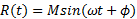With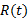the result at time t,the frequency in radians/second and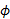the phase angle. If we want to recreate the time-history of the result, we need to plug in the magnitude and phase as reported by the FEA post-processor into the equation above and plot it over time with a spreadsheet or some other tool.

Few FEA post-processors can plot the results in this format, hence the requirement for an external tool. The reason for this seeming lack of capability is that it is a result that is almost exclusively used by novices to frequency response analysis: The more advanced users know that the original format tells them everything they need to know, and therefore no conversion from the frequency domain to the time-domain is really needed.

However, this same “missing functionality” makes it harder for Engineers starting out in frequency response analysis. Most FEA post-processors has a tool that allows you to see the result above in a more cumbersome (but very useful) way. Let’s first re-write the equation above to a more useful format.

If we recognize that the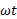term is simply an angle that increases with time, we can replace it with an angle variable. This is even more useful when we realize that a sine-wave repeat every 360 degrees (or 2π radians). The equation now becomes: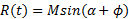Where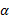is the angle in the cycle of the sinewave. By plotting results at an angle(not the same as plotting the phase angle!), we can step through the results over a cycle by varying the angle from zero degrees up to 360 degrees after which the results repeat. This is a manual process, but worth it initially to try and better understand what the results mean.

Things become a lot more complex when we have a structure where loads at multiple frequencies act simultaneously.

It is important to note that we may still be able to use Frequency Response analysis even if multiple input frequencies exist. This is because of our assumption that the structure behaves linearly. For linear systems, we can use the superposition principal which allows you to calculate the results due to different loads, then combine the results.

For a case with multiple input frequencies, we're still solving the steady-state response of each frequency separately. Once the frequency response analysis has completed, we need to combine the transient results of each over time by summing them together to get the response as part of a post-processing step. This is similar to the process we would follow in a static analysis where we can combine the results of two load-cases.

Recreating time-histories from the Frequency Response results is an intuitive way to look at results because we are used to working in the time domain. However, it is a very inefficient way of working. The reason for this has to do with what we want to learn from a frequency response: It is very seldom the time-history, but rather whether the structure will fail or what the maximum deformation will be. Let’s look at some common cases where we would perform a frequency response analysis and what we want to learn from the analyses.

Fatigue

Let’s say we want to know whether a component mounted to a structure that has random vibration input loading will fail. To do this, we need to perform a fatigue calculation on the structure. If we solve in the time-domain, an input signal representing the Power Spectral Density (PSD) curve with random phase would need to be created and a transient analysis solved. The results will be used to determine the stress history of the location(s) of interest. This stress history is then fed into a fatigue calculation tool that creates bins of stress-ranges, and for each stress-range determine the number of cycles. This is then fed into the fatigue equation to determine the damage of the structure.

However, for the case where the PSD input does not change, the transient behavior at startup will disappear over time with only the steady state response remaining. This is exactly where a frequency response analysis excels: Calculating steady state response for each input frequency. Since the output of a frequency response is magnitude and phase per frequency, the stress range (double the amplitude) per frequency is available. Using statistical methods, the same stress-range bins and number of cycles in each bin can easily be calculated. The equations for this is out of the scope of this article, but most fatigue calculation software can an input PSD and Frequency response analysis to calculate the damage per second.

This is the same result as we’d get out of a transient analysis followed by a fatigue analysis, but in a fraction of the time and with a lot less space requirements.

In other words, the purpose of the frequency response is as an intermediate step to the result that we are interested in, namely “What is the fatigue life of my component”

Rotating components

Rotating components with an unbalance such as an internal combustion engine applies a time-varying load. Some rotating components will apply the load purely at the rotating speed such as an unbalance on a spinning shaft or pump. Others, like internal combustion engines, will apply the load at the rotating speed and several harmonics of the rotating speed.

If the speed is constant, then calculating stress-ranges, vibration levels or fatigue life is easy since we only have one frequency to contend with. All the results are already reported at this frequency as a magnitude and phase.

For components that can change speed, the frequency response can be used to determine at which frequencies the structure will amplify the vibration the most. More vibration means more damage per cycle or more noise. An internal combustion engine is a great example: As the engine revs change, so does the vibration you feel in the vehicle, but also the noise level changes. Using frequency response analysis during the design phase helps the designer to determine whether there will be frequencies that will have excessive vibration or noise. The design can then be changed as needed to improve the vibration and noise characteristics of the vehicle if required.

Conclusion

The most common problems with frequency response analysis is to understand what it is used for and how to use the results. It is easy to set up the analysis once you understand what the loads are.

A frequency response analysis is performed to determine the steady state vibration for a range of frequencies, one at a time. It can be used for structures which operate continuously at a single speed or those which change speed slowly enough so that steady state is maintained. It can also be used for those that operate in a random-vibration environment where the vibration spectrum stays constant.

One common use of frequency response analysis is to plot vibration-level (displacements or accelerations) as a function of frequency. This is used to determine which frequencies cause the most vibration.

The other common use is as an input to Fatigue calculation software. Combined with a PSD, the life of the structure can be determined.

6 comments on “Frequency Response Analysis - What Is it?”

1.Gary Styger says:

Thank you Gerrit! An extremely important analysis technique that often misunderstood!

2.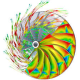jomardavalosr says:

Gerrit Whats is the i letter in the equations?Thanks in advance

1.Gerrit Visser says:

The "i" used above refers to the imaginary number: sqrt(-1)

3.cjbdl@hotmail.com says:

Awesome explanation!

4.Chetan Choudhari says:

Nicely Explained. Thanks.

5.Paul Naudé says:

yes, it's publicically available. Please credit.